Скачать презентацию Paper F 2 Management Accounting 2018 3 19 1

83a32671a93cde0ec6b66536c0c9b222.ppt

• Количество слайдов: 44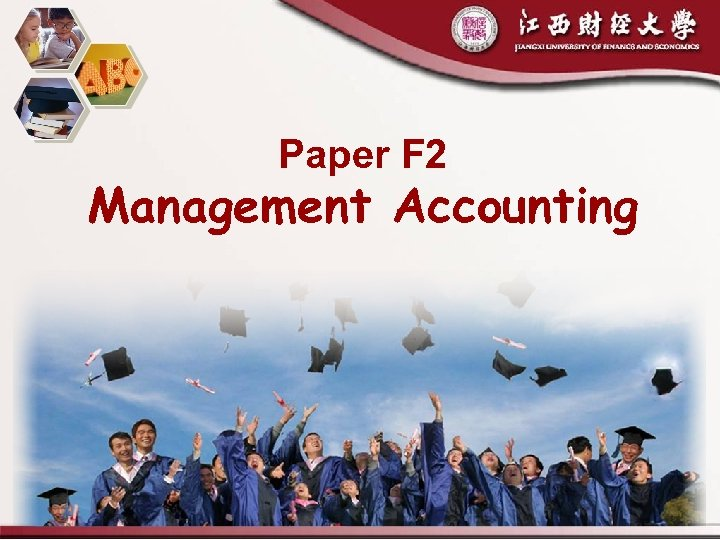Paper F 2 Management Accounting 2018/3/19 1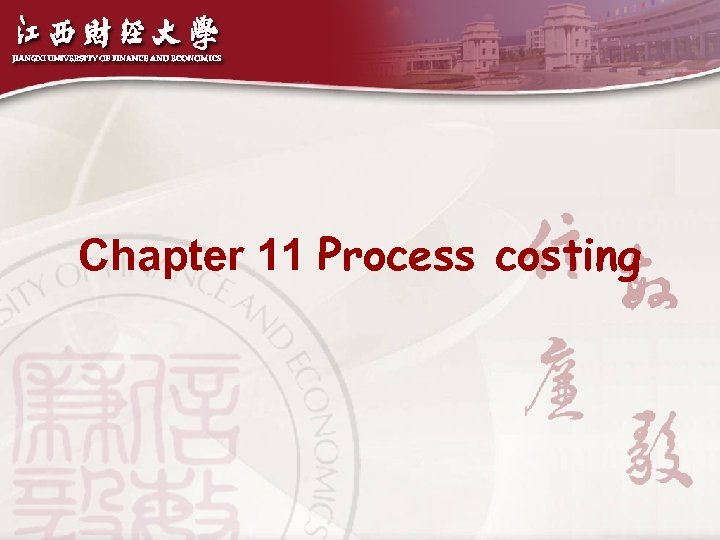Chapter 11 Process costing 2018/3/19 2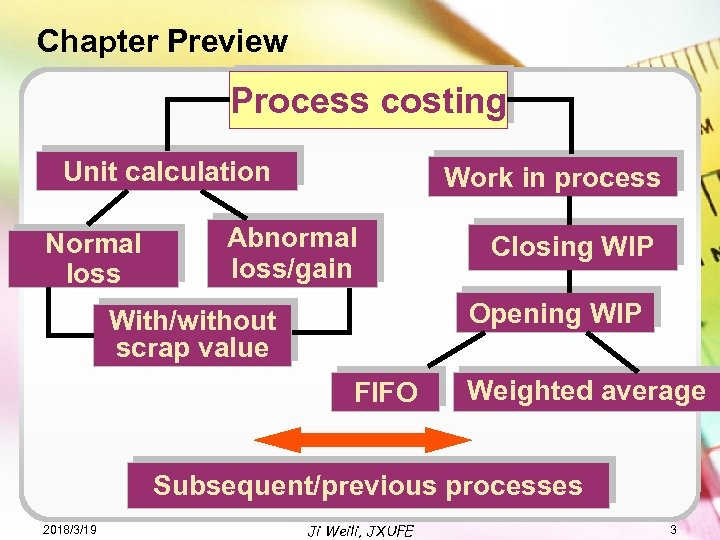Chapter Preview Process costing Unit calculation Normal loss Work in process Abnormal loss/gain Closing WIP Opening WIP With/without scrap value FIFO Weighted average Subsequent/previous processes 2018/3/19 Ji Weili, JXUFE 3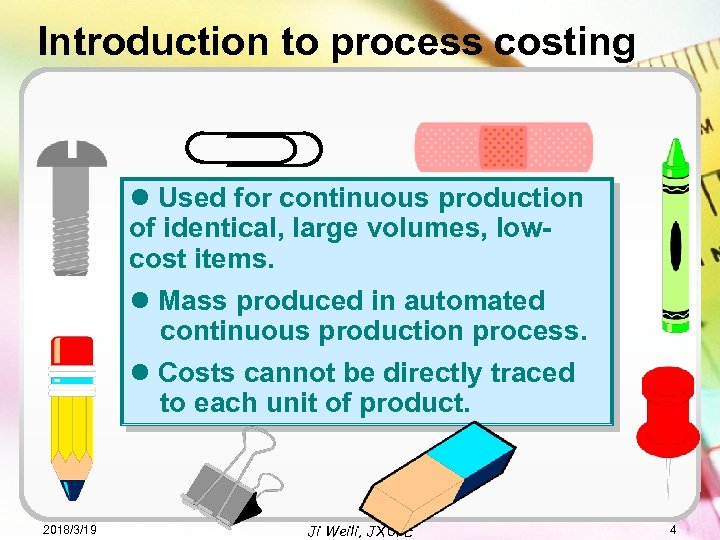Introduction to process costing l Used for continuous production of identical, large volumes, lowcost items. l Mass produced in automated continuous production process. l Costs cannot be directly traced to each unit of product. 2018/3/19 Ji Weili, JXUFE 4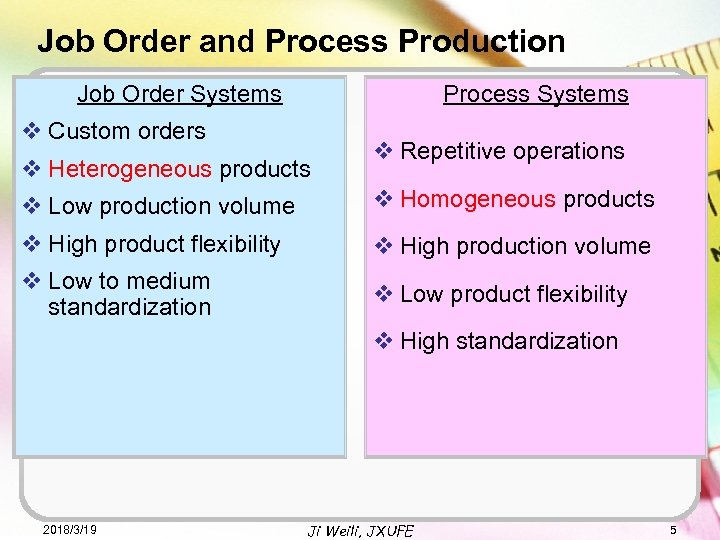Job Order and Process Production Job Order Systems Process Systems v Custom orders v Heterogeneous products v Repetitive operations v Low production volume v Homogeneous products v High product flexibility v High production volume v Low to medium standardization v Low product flexibility v High standardization 2018/3/19 Ji Weili, JXUFE 5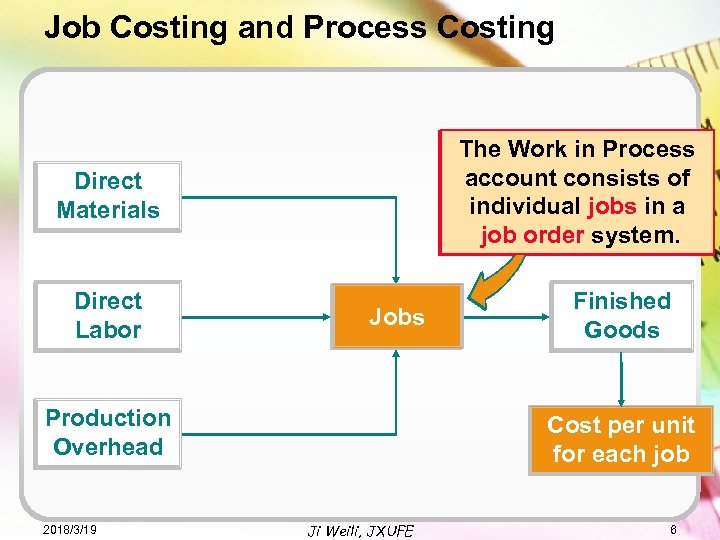Job Costing and Process Costing The Work in Process account The Work in Process consists of individual jobs in account consists of a individual system. a job order jobs in Direct Materials Direct Labor job order system. Jobs Production Overhead 2018/3/19 Finished Goods Cost per unit for each job Ji Weili, JXUFE 6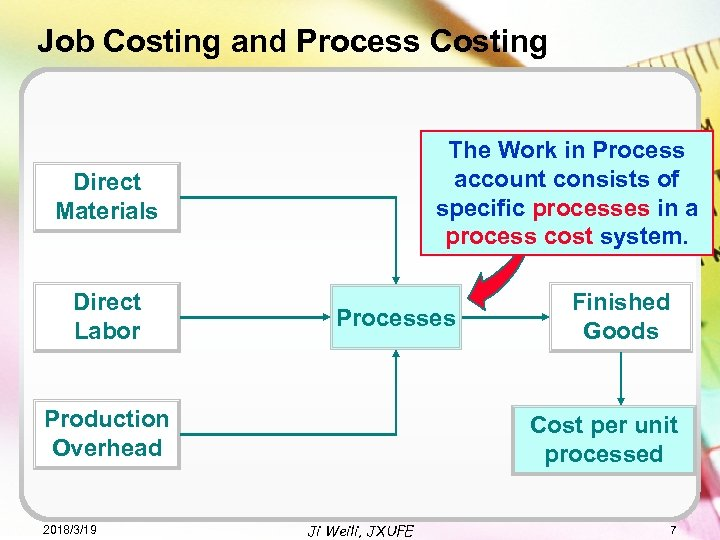Job Costing and Process Costing The Work in Process account consists of specific processes in a process cost system. Direct Materials Direct Labor Processes Production Overhead 2018/3/19 Finished Goods Cost per unit processed Ji Weili, JXUFE 7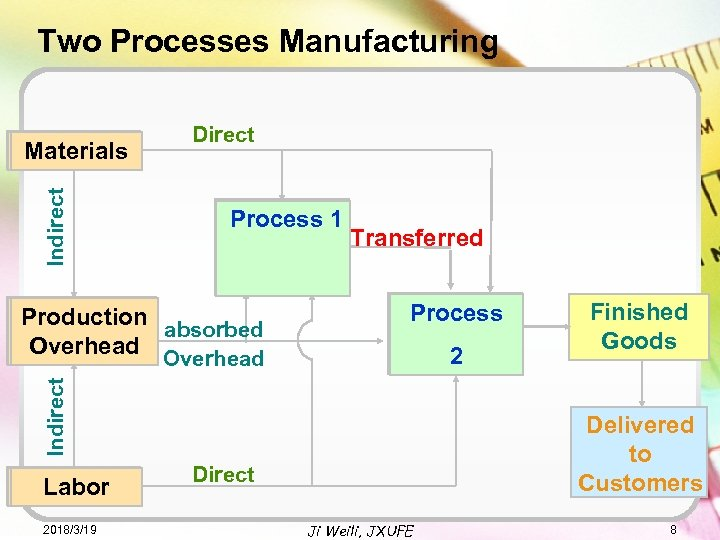Two Processes Manufacturing Indirect Materials Direct Process 1 Process 2 2 Indirect Production absorbed Overhead Transferred Labor 2018/3/19 Finished Goods Delivered to Customers Direct Ji Weili, JXUFE 8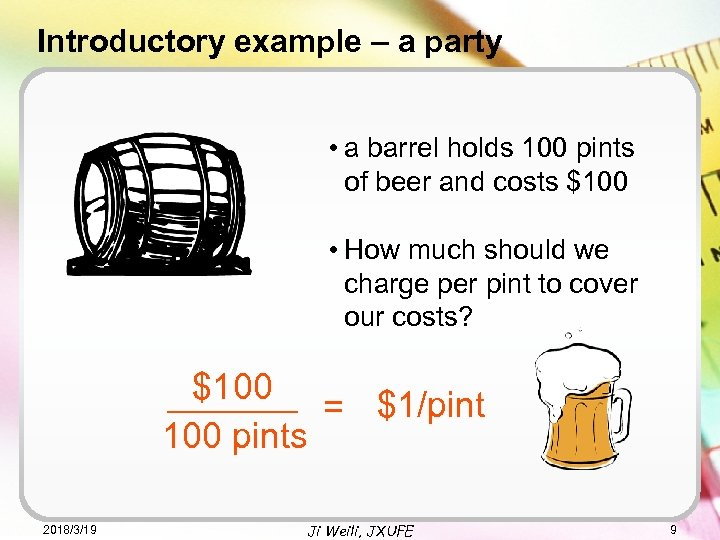Introductory example – a party • a barrel holds 100 pints of beer and costs \$100 • How much should we charge per pint to cover our costs? \$100 = \$1/pint 100 pints 2018/3/19 Ji Weili, JXUFE 9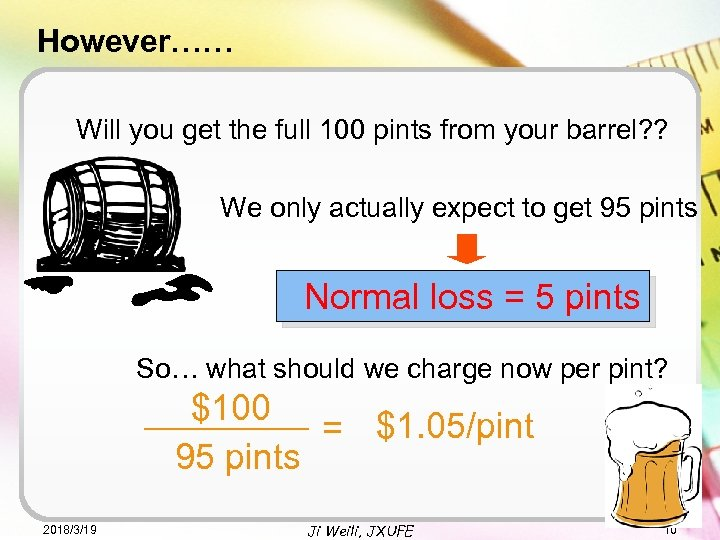However…… Will you get the full 100 pints from your barrel? ? We only actually expect to get 95 pints Normal loss = 5 pints So… what should we charge now per pint? \$100 = \$1. 05/pint 95 pints 2018/3/19 Ji Weili, JXUFE 10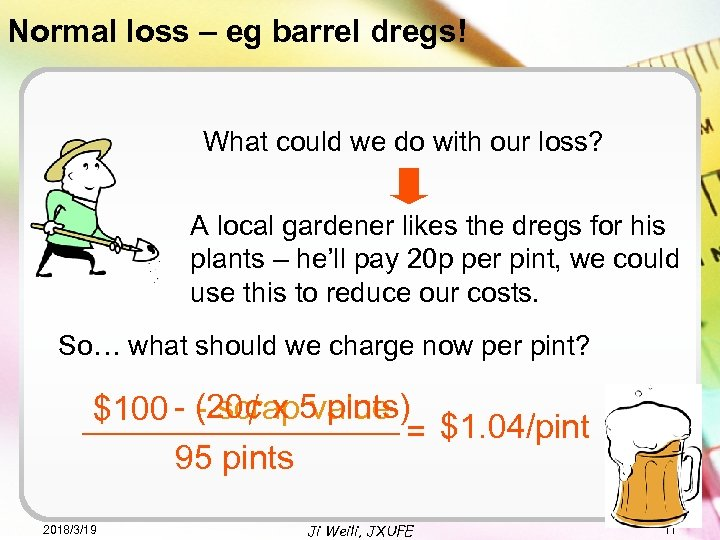Normal loss – eg barrel dregs! What could we do with our loss? A local gardener likes the dregs for his plants – he’ll pay 20 p per pint, we could use this to reduce our costs. So… what should we charge now per pint? - scrap value \$100 - (20¢ x 5 pints) = \$1. 04/pint 95 pints 2018/3/19 Ji Weili, JXUFE 11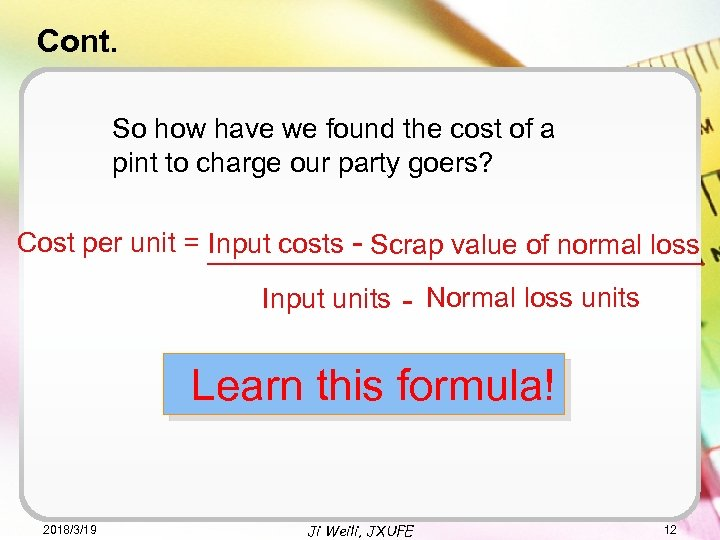Cont. So how have we found the cost of a pint to charge our party goers? Cost per unit = ________________ Input costs - Scrap value of normal loss Input units - Normal loss units Learn this formula! 2018/3/19 Ji Weili, JXUFE 12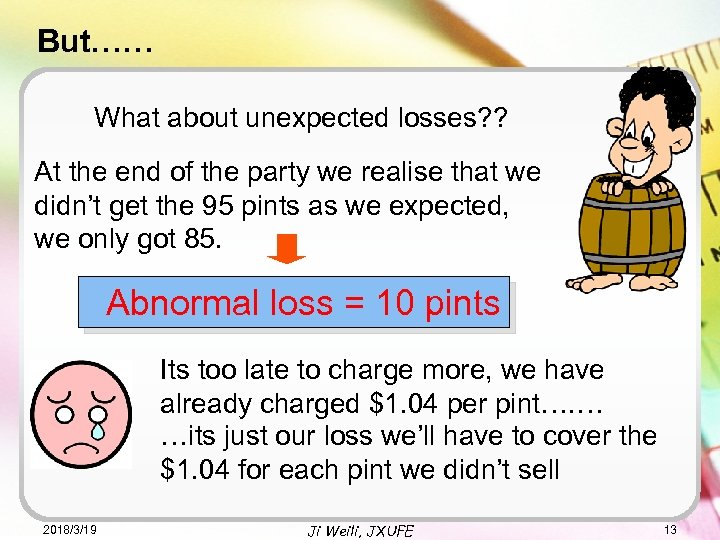But…… What about unexpected losses? ? At the end of the party we realise that we didn’t get the 95 pints as we expected, we only got 85. Abnormal loss = 10 pints Its too late to charge more, we have already charged \$1. 04 per pint…. … …its just our loss we’ll have to cover the \$1. 04 for each pint we didn’t sell 2018/3/19 Ji Weili, JXUFE 13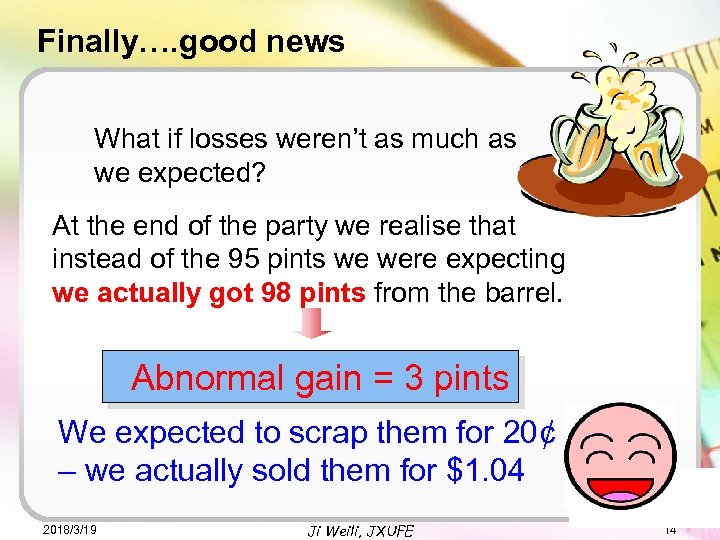Finally…. good news What if losses weren’t as much as we expected? At the end of the party we realise that instead of the 95 pints we were expecting we actually got 98 pints from the barrel. Abnormal gain = 3 pints We expected to scrap them for 20¢ – we actually sold them for \$1. 04 2018/3/19 Ji Weili, JXUFE 14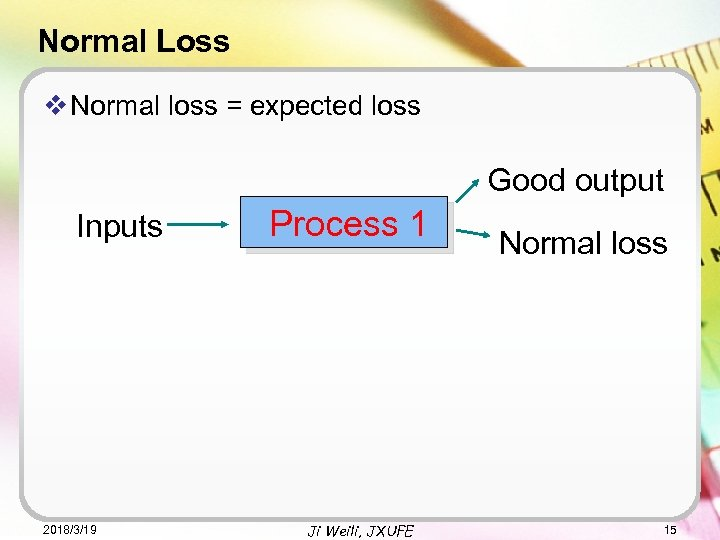Normal Loss v Normal loss = expected loss Good output Inputs 2018/3/19 Process 1 Ji Weili, JXUFE Normal loss 15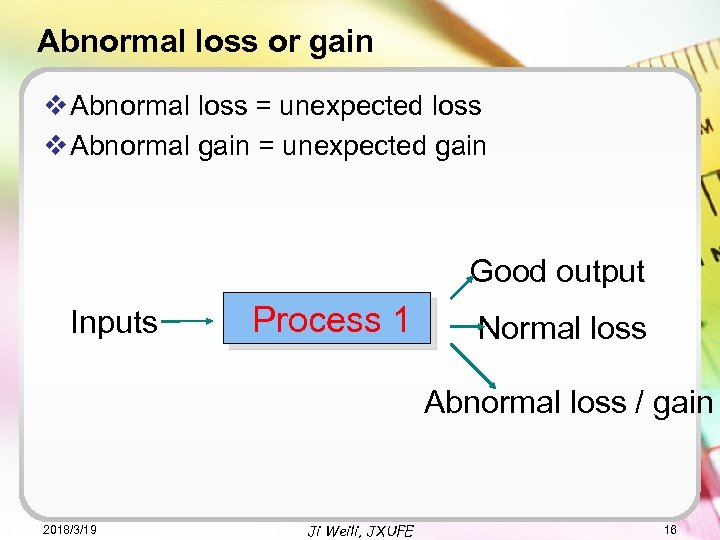Abnormal loss or gain v Abnormal loss = unexpected loss v Abnormal gain = unexpected gain Good output Inputs Process 1 Normal loss Abnormal loss / gain 2018/3/19 Ji Weili, JXUFE 16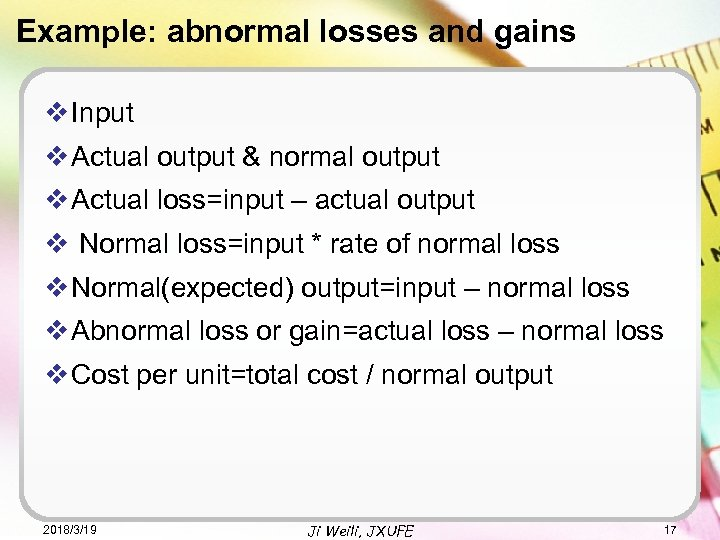Example: abnormal losses and gains v Input v Actual output & normal output v Actual loss=input – actual output v Normal loss=input * rate of normal loss v Normal(expected) output=input – normal loss v Abnormal loss or gain=actual loss – normal loss v Cost per unit=total cost / normal output 2018/3/19 Ji Weili, JXUFE 17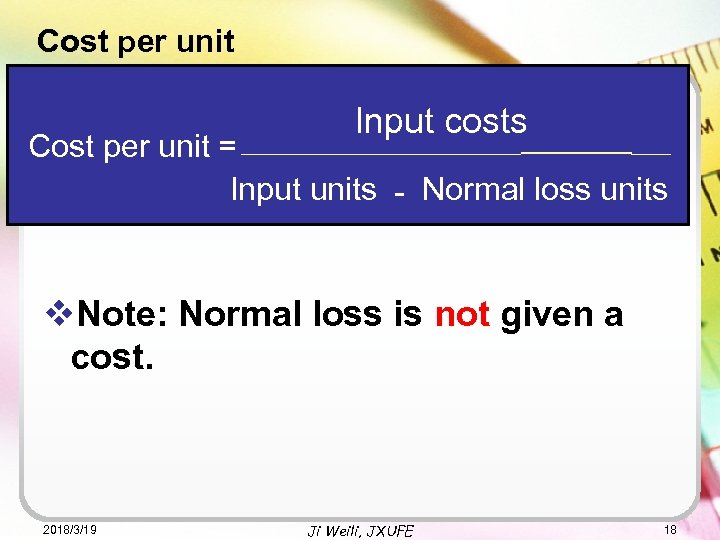Cost per unit Input costs ______________ Cost per unit = Input units - Normal loss units v. Note: Normal loss is not given a cost. 2018/3/19 Ji Weili, JXUFE 18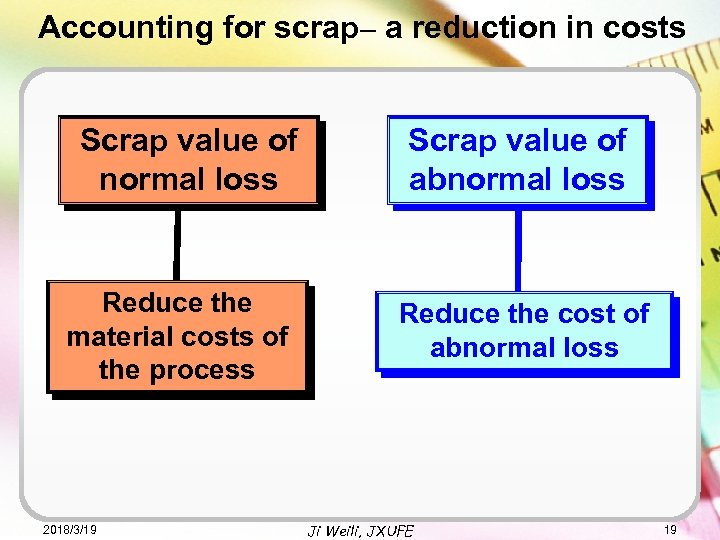Accounting for scrap– a reduction in costs Scrap value of normal loss Reduce the material costs of the process 2018/3/19 Scrap value of abnormal loss Reduce the cost of abnormal loss Ji Weili, JXUFE 19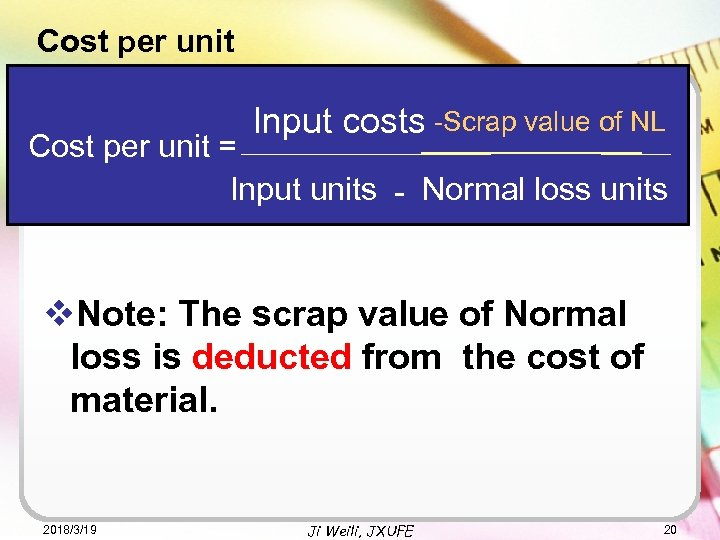Cost per unit Input costs -Scrap value of NL _____________ Cost per unit = Input units - Normal loss units v. Note: The scrap value of Normal loss is deducted from the cost of material. 2018/3/19 Ji Weili, JXUFE 20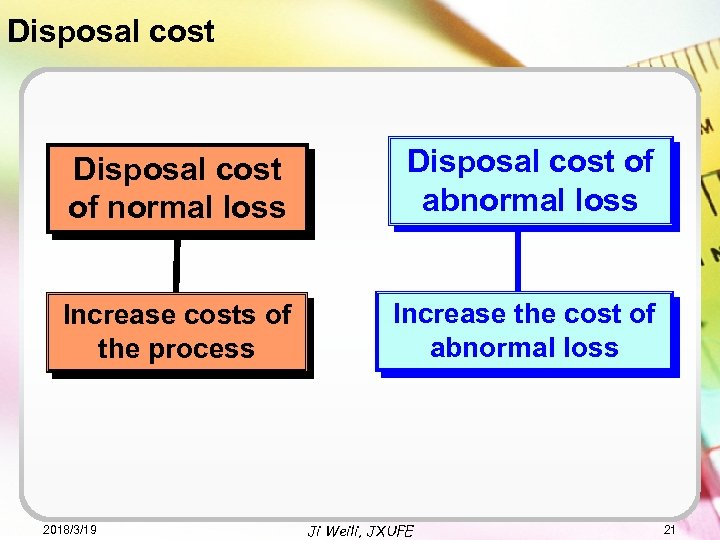Disposal cost of normal loss Disposal cost of abnormal loss Increase costs of the process Increase the cost of abnormal loss 2018/3/19 Ji Weili, JXUFE 21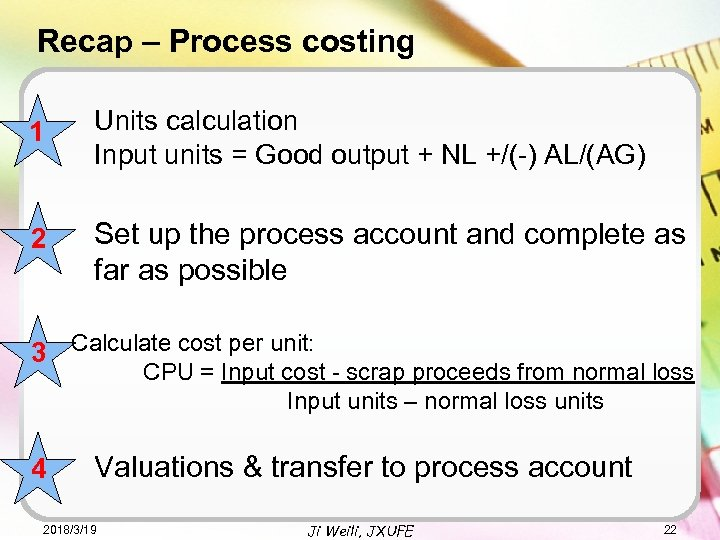Recap – Process costing 1 Units calculation Input units = Good output + NL +/(-) AL/(AG) 2 Set up the process account and complete as far as possible 3 Calculate cost per unit: CPU = Input cost - scrap proceeds from normal loss Input units – normal loss units 4 Valuations & transfer to process account 2018/3/19 Ji Weili, JXUFE 22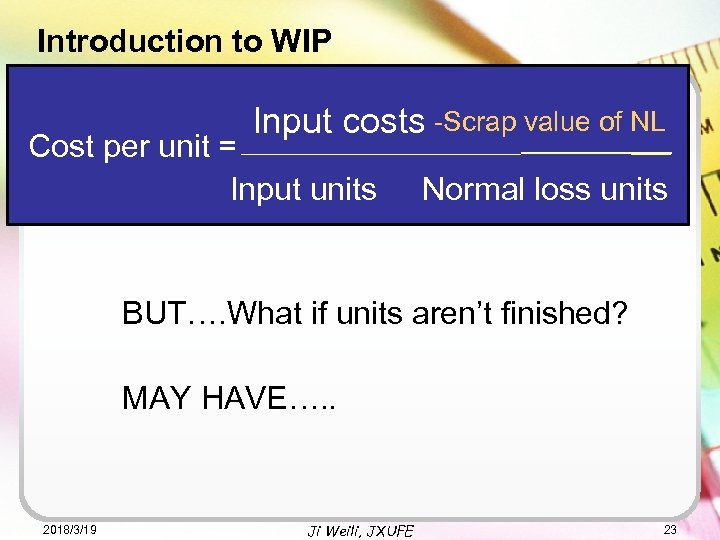Introduction to WIP Input costs -Scrap value of NL ______________ Cost per unit =________________ loss Input costs - Scrap value of normal Cost per unit = Input units Normal loss units Input units - BUT…. What if units aren’t finished? MAY HAVE…. . 2018/3/19 Ji Weili, JXUFE 23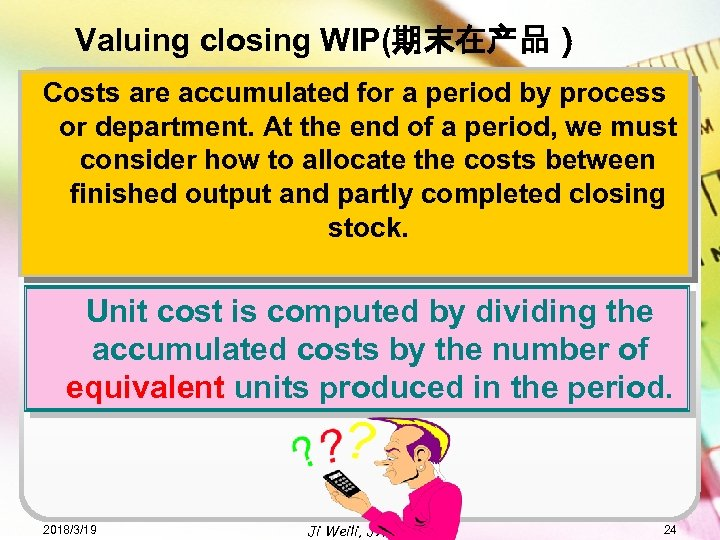Valuing closing WIP(期末在产品） Costs are accumulated for a period by process or department. At the end of a period, we must consider how to allocate the costs between finished output and partly completed closing stock. Unit cost is computed by dividing the accumulated costs by the number of equivalent units produced in the period. 2018/3/19 Ji Weili, JXUFE 24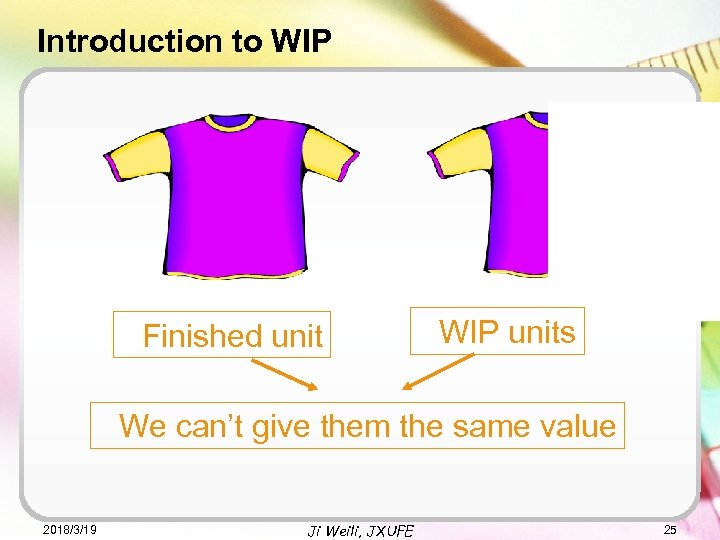Introduction to WIP Finished unit WIP units We can’t give them the same value 2018/3/19 Ji Weili, JXUFE 25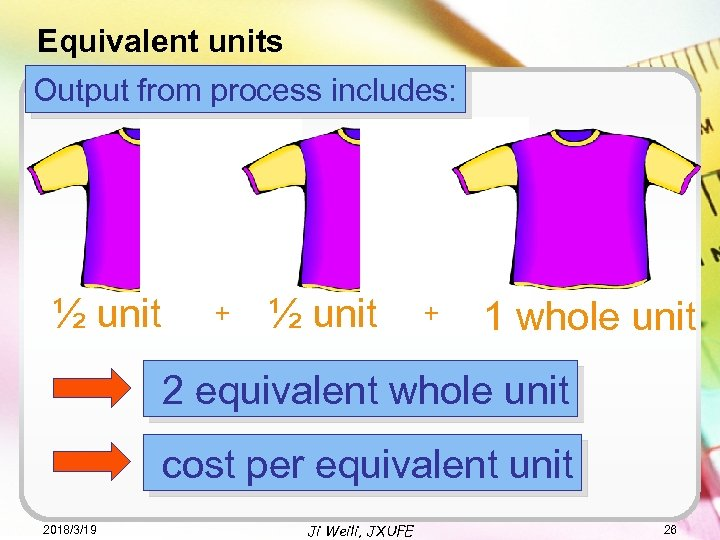Equivalent units Output from process includes: ½ unit + 1 whole unit 2 equivalent whole unit cost per equivalent unit 2018/3/19 Ji Weili, JXUFE 26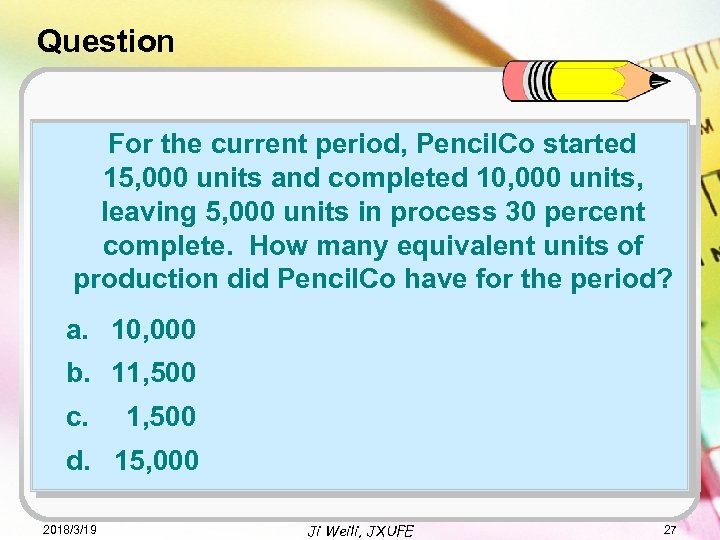Question For the current period, Pencil. Co started 15, 000 units and completed 10, 000 units, leaving 5, 000 units in process 30 percent complete. How many equivalent units of production did Pencil. Co have for the period? a. 10, 000 b. 11, 500 c. 1, 500 d. 15, 000 2018/3/19 Ji Weili, JXUFE 27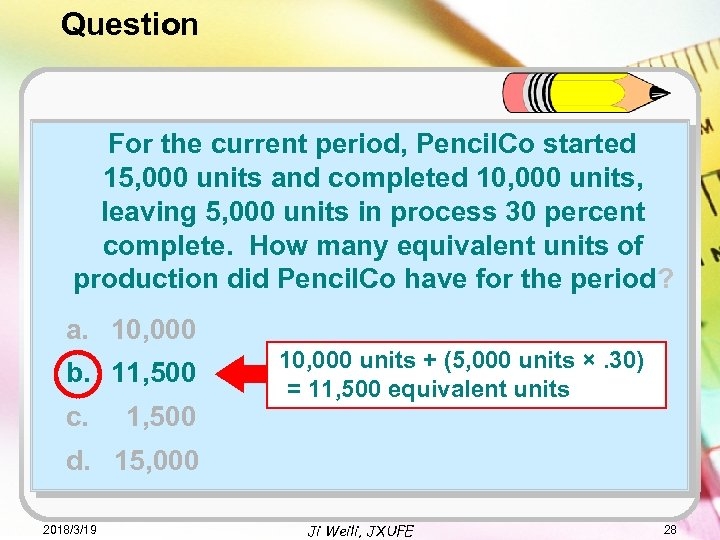Question For the current period, Pencil. Co started 15, 000 units and completed 10, 000 units, leaving 5, 000 units in process 30 percent complete. How many equivalent units of production did Pencil. Co have for the period? a. 10, 000 b. 11, 500 c. 1, 500 10, 000 units + (5, 000 units ×. 30) = 11, 500 equivalent units d. 15, 000 2018/3/19 Ji Weili, JXUFE 28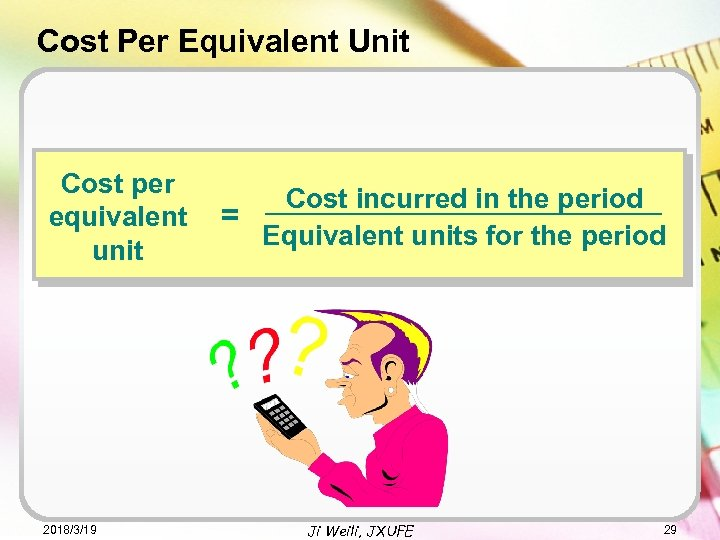Cost Per Equivalent Unit Cost per equivalent unit 2018/3/19 Cost incurred in the period = Equivalent units for the period Ji Weili, JXUFE 29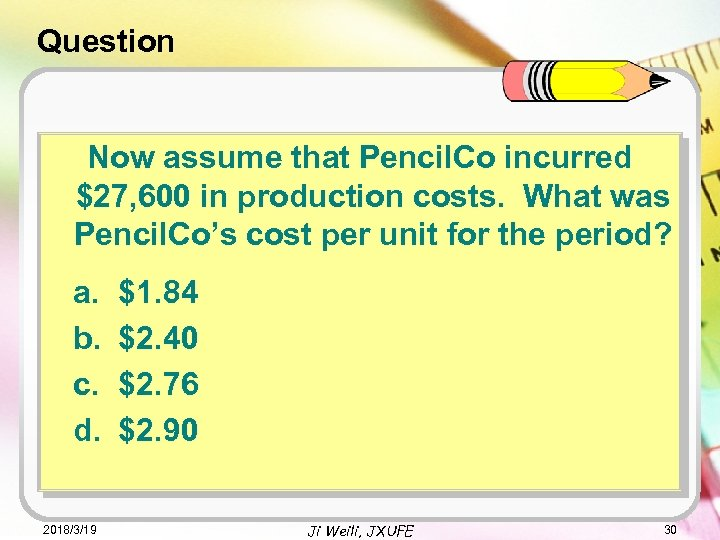Question Now assume that Pencil. Co incurred \$27, 600 in production costs. What was Pencil. Co’s cost per unit for the period? a. b. c. d. 2018/3/19 \$1. 84 \$2. 40 \$2. 76 \$2. 90 Ji Weili, JXUFE 30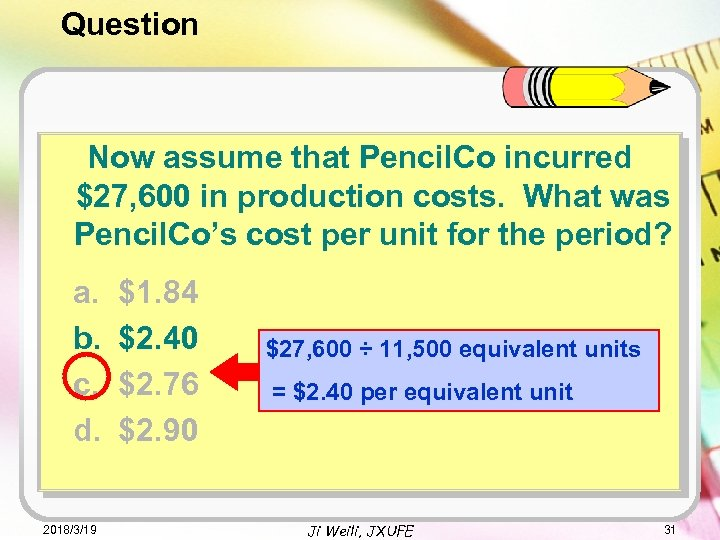Question Now assume that Pencil. Co incurred \$27, 600 in production costs. What was Pencil. Co’s cost per unit for the period? a. b. c. d. 2018/3/19 \$1. 84 \$2. 40 \$2. 76 \$2. 90 \$27, 600 ÷ 11, 500 equivalent units = \$2. 40 per equivalent unit Ji Weili, JXUFE 31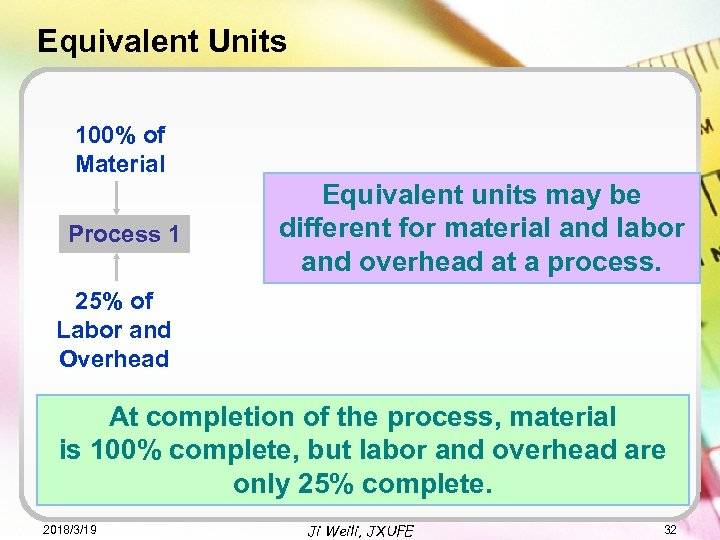Equivalent Units 100% of Material Process 1 Equivalent units may be different for material and labor and overhead at a process. 25% of Labor and Overhead At completion of the process, material is 100% complete, but labor and overhead are only 25% complete. 2018/3/19 Ji Weili, JXUFE 32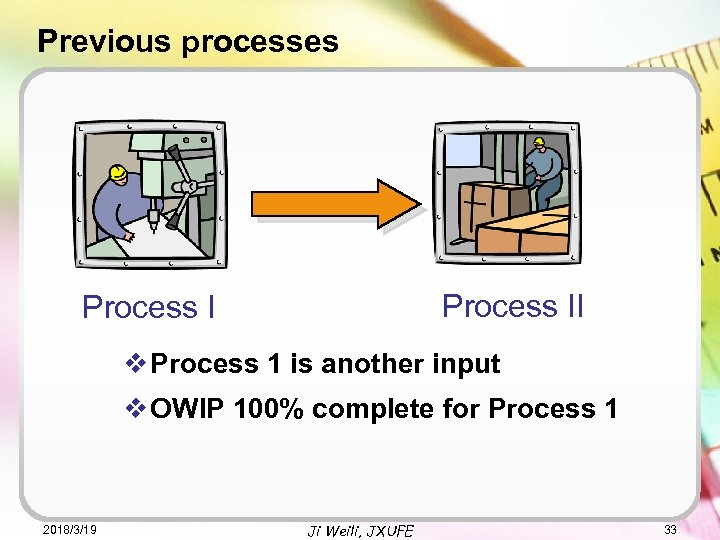Previous processes Process II Process I v Process 1 is another input v OWIP 100% complete for Process 1 2018/3/19 Ji Weili, JXUFE 33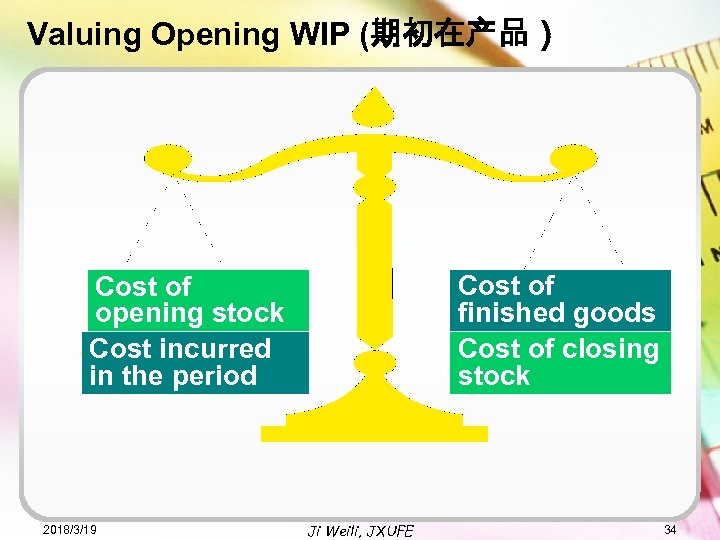Valuing Opening WIP (期初在产品） Cost of finished goods Cost of closing stock Cost of opening stock Cost incurred in the period 2018/3/19 Ji Weili, JXUFE 34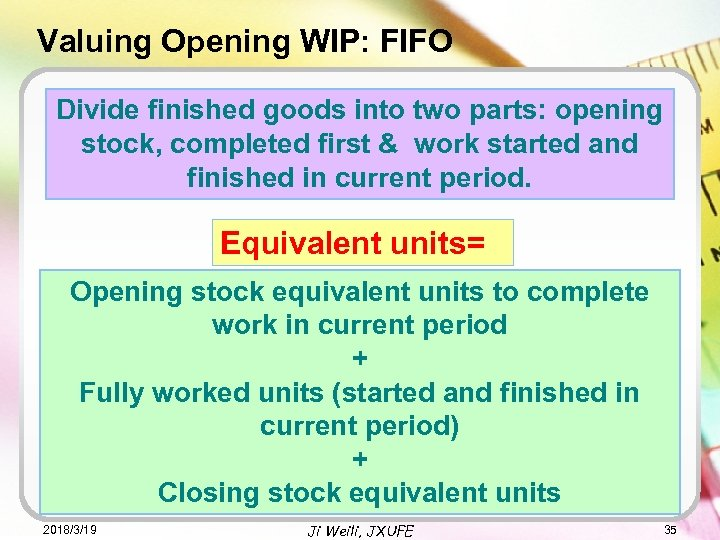Valuing Opening WIP: FIFO Divide finished goods into two parts: opening stock, completed first & work started and finished in current period. Equivalent units= Opening stock equivalent units to complete work in current period + Fully worked units (started and finished in current period) + Closing stock equivalent units 2018/3/19 Ji Weili, JXUFE 35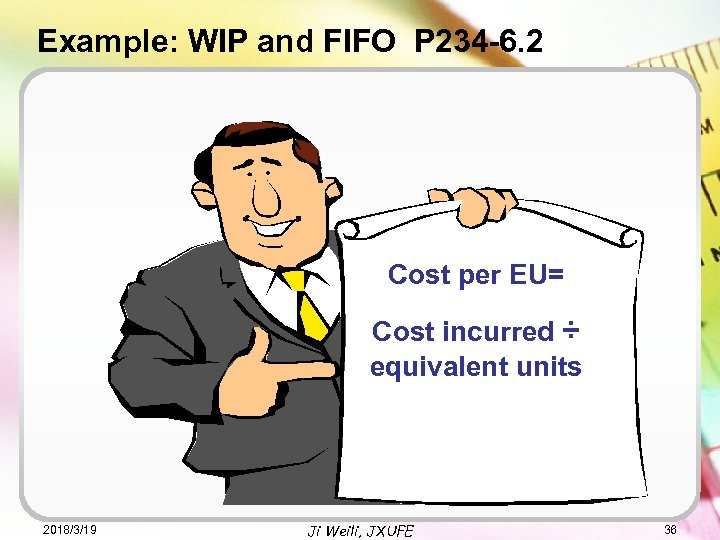Example: WIP and FIFO P 234 -6. 2 Cost per EU= Cost incurred ÷ equivalent units 2018/3/19 Ji Weili, JXUFE 36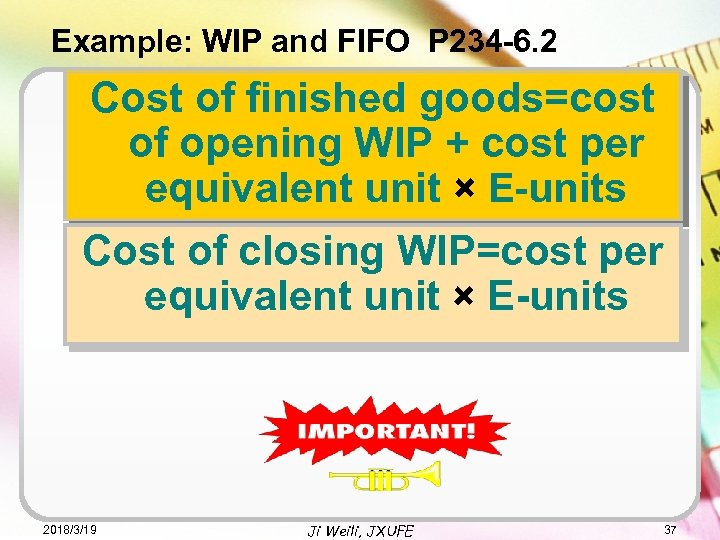Example: WIP and FIFO P 234 -6. 2 Cost of finished goods=cost of opening WIP + cost per equivalent unit × E-units Cost of closing WIP=cost per equivalent unit × E-units 2018/3/19 Ji Weili, JXUFE 37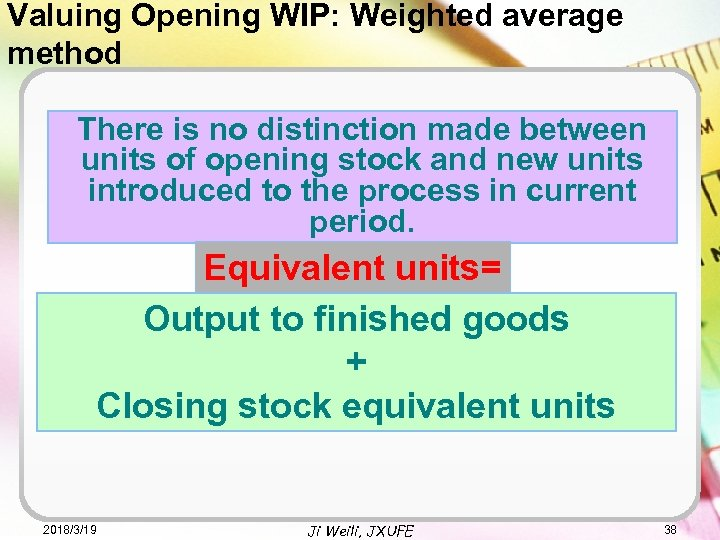Valuing Opening WIP: Weighted average method There is no distinction made between units of opening stock and new units introduced to the process in current period. Equivalent units= Output to finished goods + Closing stock equivalent units 2018/3/19 Ji Weili, JXUFE 38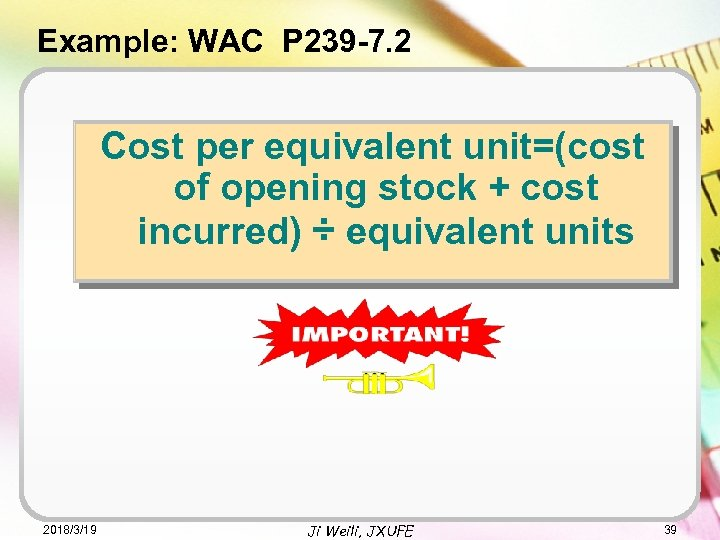Example: WAC P 239 -7. 2 Cost per equivalent unit=(cost of opening stock + cost incurred) ÷ equivalent units 2018/3/19 Ji Weili, JXUFE 39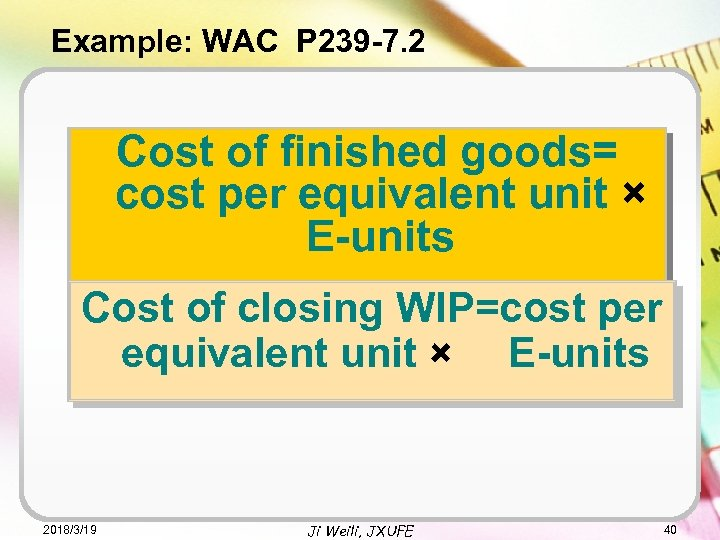Example: WAC P 239 -7. 2 Cost of finished goods= cost per equivalent unit × E-units Cost of closing WIP=cost per equivalent unit × E-units 2018/3/19 Ji Weili, JXUFE 40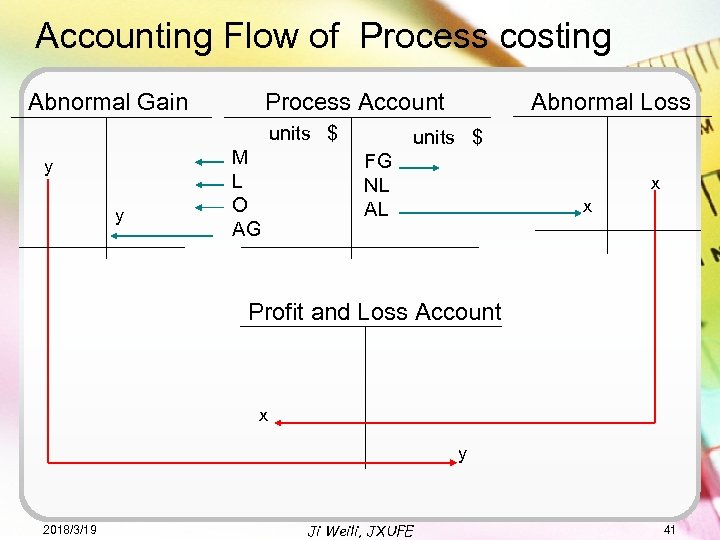Accounting Flow of Process costing Abnormal Gain units \$ y y Abnormal Loss Process Account M L O AG units \$ FG NL AL x x Profit and Loss Account x y 2018/3/19 Ji Weili, JXUFE 41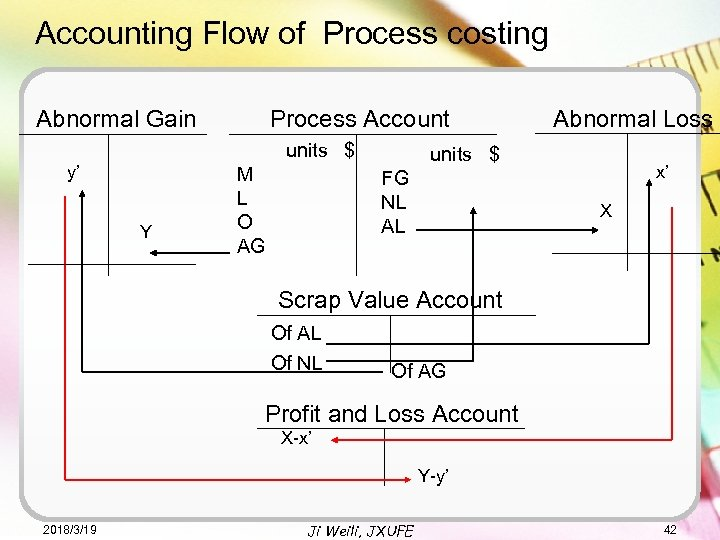Accounting Flow of Process costing Abnormal Gain Process Account units \$ y’ Y M L O AG Abnormal Loss units \$ FG NL AL x’ X Scrap Value Account Of AL Of NL Of AG Profit and Loss Account X-x’ Y-y’ 2018/3/19 Ji Weili, JXUFE 42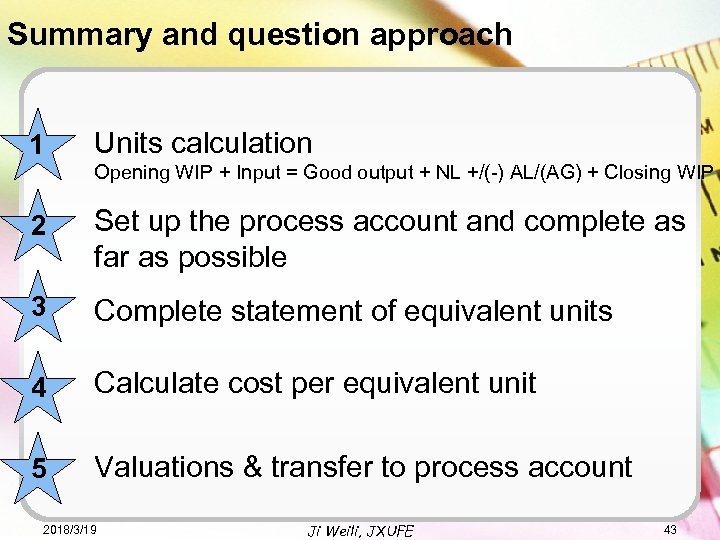Summary and question approach 1 Units calculation Opening WIP + Input = Good output + NL +/(-) AL/(AG) + Closing WIP 2 Set up the process account and complete as far as possible 3 Complete statement of equivalent units 4 Calculate cost per equivalent unit 5 Valuations & transfer to process account 2018/3/19 Ji Weili, JXUFE 43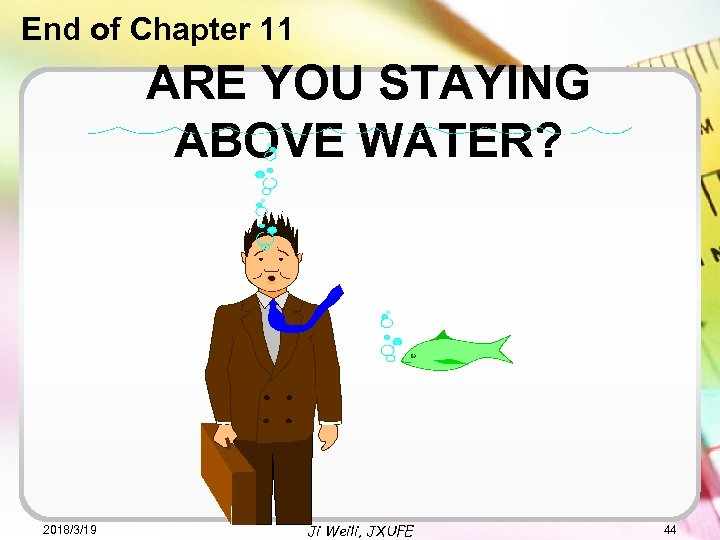End of Chapter 11 ARE YOU STAYING ABOVE WATER? 2018/3/19 Ji Weili, JXUFE 44